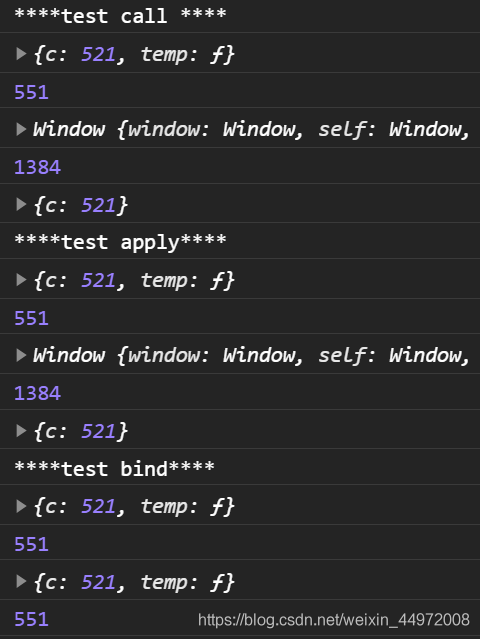【JS】JavaScript基础知识自我检查大过关（第三关）函数定义与调用

theme: fancy highlight: a11y-dark

「这是我参与11月更文挑战的第28天，活动详情查看：2021最后一次更文挑战

1. 函数定义的几种方式

1.1 函数声明式

javascript function fn(a, b) { return a + b; }

1.2 函数表达式

javascript let fun = function(a, b){ return a + b; }

1.3 构造函数式

javascript let fun = new Function('a', 'b', 'return a + b')

1.4 箭头函数

javascript let fn = (a, b) => { return a + b; }; 关于箭头函数的更多内容，请见【ES6】JavaScript函数-箭头函数-this指向-简写

2. 函数调用的几种方式

2.1 普通函数

javascript fun() fun.call()

2.2 对象的方法

javascript obj.fun()

2.3 构造函数

javascript new Fun()

4. 改变函数的this指向

4.1 使用call方法

javascript fun.call(thisArg, arg1, arg2, ...)

```javascript function add(a, b) { console.log(a + b) }

let obj = { c: 520 } add(1,2) // this指向window add.call(obj, 1, 2) // this指向obj ```

应用

```javascript function Father(uname, age, sex) { this.uname = uname this.age = age this.sex = sex }

function Son(uname, age, sex){ Father.call(this, uname, age, sex) }

let son = new Son('YK菌', 18, '男') console.log(son) // Son {uname: "YK菌", age: 18, sex: "男"} ```

4.2 使用apply方法

javascript fun.apply(thisArg, [argsArray])

let obj = { c: 520 } add(1,2) // this指向window add.call(obj, [1, 2]) // this指向obj ```

应用

javascript let arr = [1, 34, 556, 44, 23] Math.max.apply(null, arr); // null 表示不需要改变this指向 Math.max.apply(Math, arr); // 不改变的话最好就指回去

4.3 使用bind方法

javascript fun.bind(thisArg, arg1, arg2, ...)

```javascript function add(a, b) { console.log(a + b) }

let obj = { c: 520 } add(1,2) // this指向window let f = add.bind(obj, 1, 2) // this指向obj， 不会调用 f() // 调用函数 this指向obj ```

应用

javascript let btn = document.querySelector('button') btn.onclick = function() { this.disabled = true setTimeout(function(){ this.disabled = false; }.bind(this), 3000) }

4.4 区别与联系

区别

callapply 会调用函数, 并且改变函数内部this指向. callapply 传递的参数不一样, call 传递参数 arg1, arg2...形式 apply 必须数组形式[args] bind 不会调用函数, 可以改变函数内部this指向.

主要应用场景

call 经常做继承. apply 经常跟数组有关系. 比如借助于数学对象实现数组最大值最小值 bind 不调用函数,但是还想改变this指向. 比如改变定时器内部的this指向

5. 自定义call、apply、bind方法

call.js

javascript export default function call(Fn, obj, ...args) { if (obj === undefined || obj === null) { // 表示全局对象（ES11新增特性） obj = globalThis; } // 为 obj 添加临时的方法 obj.temp = Fn; // 调用 temp 方法 let result = obj.temp(...args); // 删除tempfangfa delete obj.temp; // 返回执行结果 return result; }

apply.js

```javascript export default function apply(Fn, obj, arr) { if (obj === undefined || obj === null) { obj = globalThis; } // 为obj添加临时方法 obj.temp = Fn; // 执行方法 let result = obj.temp(...arr); // 删除临时属性 delete obj.temp; // 返回结果 return result; }

```

bind.js

```javascript import call from './call.js'

export default function bind(Fn, obj, ...args) { // 返回一个新的函数 return function (...args2) { // 执行 call 函数、 return call(Fn, obj, ...args, ...args2); }; }

```

测试

```javascript import call from "./function/call"; import apply from "./function/apply"; import bind from "./function/bind";

console.log("*test call *");

function add(a, b) { console.log(this); return a + b + this.c; } let obj = { c: 521, }; window.c = 1314;

let fn2 = bind(add, obj); console.log(fn2(10, 20)); ```